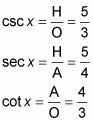##### Trigonometry For Dummies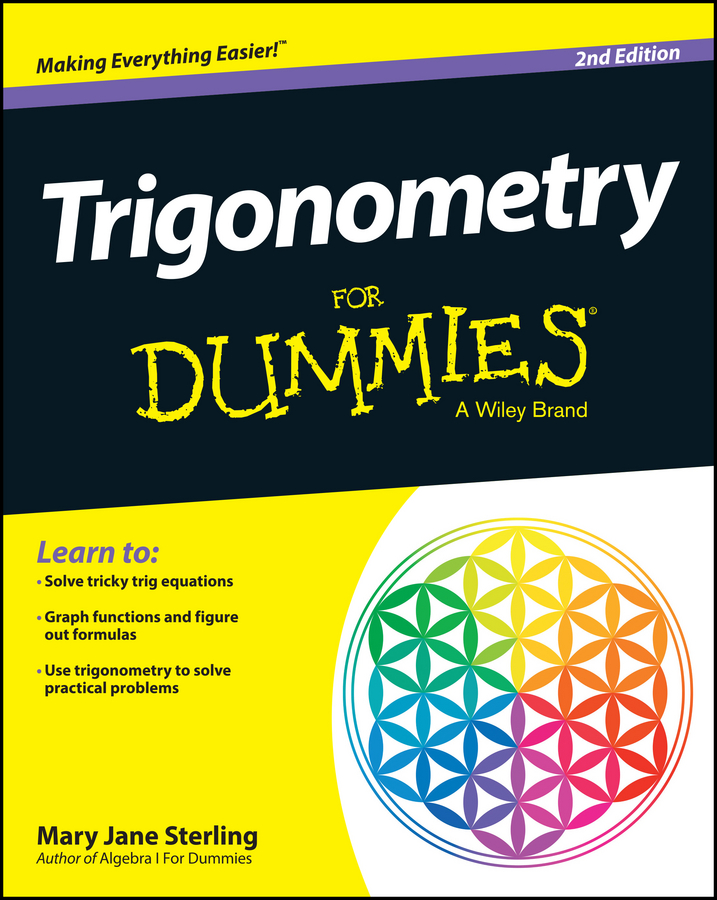The study of trigonometry begins with the right triangle. The three main trig functions (sine, cosine, and tangent) and their reciprocals (cosecant, secant, and cotangent) all tell you something about the lengths of the sides of a right triangle that contains a given acute angle — like angle x in the figure below.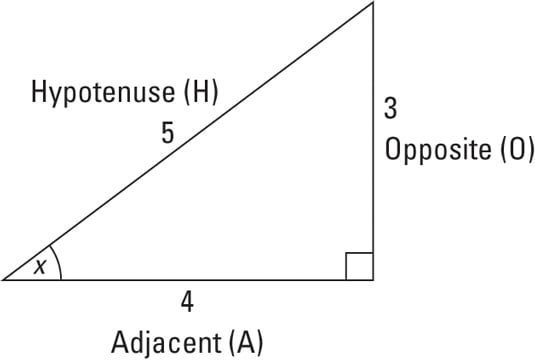The longest side of this right triangle (or any right triangle), the diagonal side, is called the hypotenuse. The side that’s 3 units long is referred to as the opposite side because it’s on the opposite side of the triangle from angle x, and the side of length 4 is called the adjacent side because it’s adjacent to, or touching, angle x.

SohCahToa is a meaningless mnemonic that helps you remember the definitions of the sine, cosine, and tangent functions. SohCahToa uses the initial letters of sine, cosine, and tangent, and the initial letters of hypotenuse, opposite, and adjacent, to help you remember the following definitions. (To remember how to spell SohCahToa, note its pronunciation and the fact that it contains three groups of three letters each.)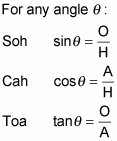For the triangle in the preceding figure: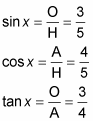The other three trig functions are reciprocals of these: Cosecant (csc) is the reciprocal of sine, secant (sec) is the reciprocal of cosine, and cotangent (cot) is the reciprocal of tangent.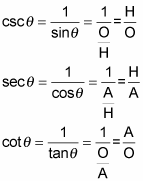For the triangle in the preceding figure: